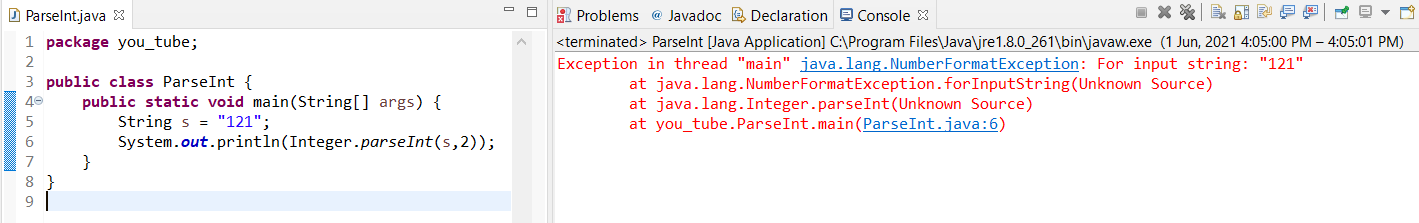The parseint Java method is used to simply convert any String to Primitive int type.

An int basically means Integer (…-3,-2,-1,0,1,2,3…) with a range of -2147483648 to 2147483647.

Syntax:

1. `Integer.parseInt(String mydata);`

2. `Integer.parseInt(String mydata, int radix);`

In a shell, there are two Java syntaxes to use `parseInt()` method. It takes in the provided String data as the first parameter. If a second parameter is provided it should be of int type denoting the radix.

A radix is the base of a number. For instance, 2 means Binary, 8 means Octal, 10 is Decimal and 16 is hexadecimal. Imagine radix as the number of unique digits one can use including 0 to represent numbers.

### Example 1

Convert a String to Integer and return the square of the number.

Solution

``````public class ParseInt {
public static void main(String[] args) {
String s = "11";
int i = Integer.parseInt(s); // conversion
int result = i*i;
System.out.println("The square of the number is: "+result);
}
}``````

Output
`The square of the number is: 121`

### Java parseint Example 2

In a school, a student with id 1 has left. Now modify all the student ids(reduce by 1). You are given a String of a student name with the last 2 characters as id number. Extract the id number. Display the name with the correct id.

Sample
`input: John65 Output: name-John roll-64`

`input: Ana03 Output: name-Ana roll-2`

Solution

``````public class ParseInt {
public static void main(String[] args) {
String s = "John65";
String name = s.substring(0, s.length()-2);//extract name
String id_string = s.substring(s.length()-2);//extract id
int id_int = Integer.parseInt(id_string);//convert id from String to int
int correct_id = id_int-1;//correct id
System.out.println("name-"+name+" roll-"+correct_id);//print output
}
}
``````

Output
`name-John roll-64`

### Exceptions in parseint Java

NumberFormatException is generally thrown by the `parseInt()` method in Java, if any of the following cases occur:

1. The string is null or empty
2. In addition, The value in the string is not an integer
3. Specifically for the `parseInt(String s, int radix)` a variant of the function, if the number contains digits outside the range of the specified radix. For instance, if we define radix as 2 and pass “101” and “121”. Clearly, radix 2 means it is a Binary number. We know that 121 is not a valid Binary number. Let’s code and see.

Valid Case – Example Program

``````public class ParseInt {
public static void main(String[] args) {
String s = "101";
System.out.println(Integer.parseInt(s,2));
}
}
``````

OutputValid Output of parseInt() Java

Invalid Case – Example Program

``````public class ParseInt {
public static void main(String[] args) {
String s = "121";
System.out.println(Integer.parseInt(s,2));
}
}
``````

OutputInvalid Output of parseInt() Java

Let’s say we want to handle the above exception. Instead of getting the entire program to a halt, we want to tell the users about the problem.

The exercise is simple. If a NumberFormatException occurs, tell the user that it is not a valid number!

Great if you tried! Here’s the solution for help.

code

``````public class ParseInt {
public static void main(String[] args) {
try {
String s = "%101"; // invalid number
System.out.println(Integer.parseInt(s));
}
catch(NumberFormatException e) {
System.out.println("not a valid number!");
}
}
}
``````

Output
`not a valid number!`

Finally, We have used a try-catch block. If a NumberFormatException occurs the catch block is executed. Then we can tell users about the exception.

Likewise, You can also read out popular posts on int to String Java – 5 Ways to Convert int to String in Java

We hope this tutorial helped how to use parseint Java and you learned what does parseint do in Java? Keep learning keep sharing. Follow us on Facebook and Instagram.